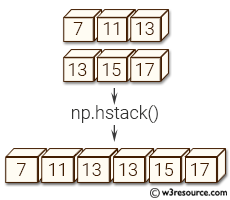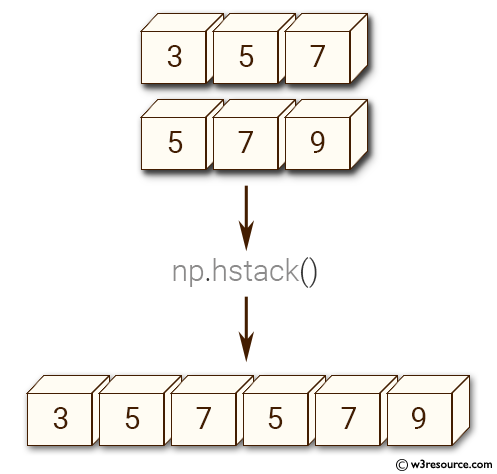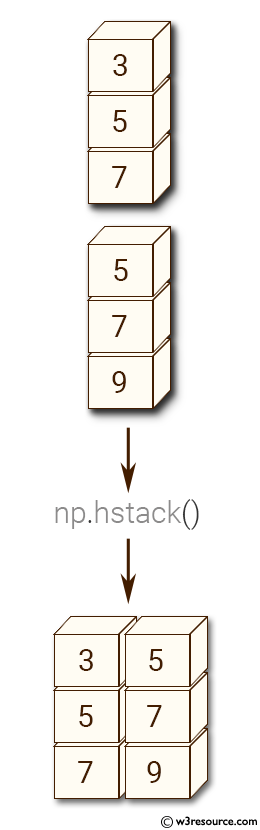# NumPy Array manipulation: hstack() function

## numpy.hstack() function

The hstack() function is used to stack arrays in sequence horizontally (column wise).
This is equivalent to concatenation along the second axis, except for 1-D arrays where it concatenates along the first axis. Rebuilds arrays divided by hsplit.
This function makes most sense for arrays with up to 3 dimensions. For instance, for pixel-data with a height (first axis), width (second axis), and r/g/b channels (third axis). The functions concatenate, stack and block provide more general stacking and concatenation operations.

Syntax:

`numpy.hstack(tup)`Version: 1.15.0

Parameter:

Name Description Required /
Optional
tup The arrays must have the same shape along all but the second axis, except 1-D arrays which can be any length. Required

Return value:

stacked : ndarray The array formed by stacking the given arrays.

Example-1: numpy.hstack()

``````>>> import numpy as np
>>> x = np.array((3,5,7))
>>> y = np.array((5,7,9))
>>> np.hstack((x,y))
array([3, 5, 7, 5, 7, 9])
``````

Pictorial Presentation:Example-2: numpy.hstack()

``````>>> import numpy as np
>>> x = np.array([, , ])
>>> y = np.array([, , ])
>>> np.hstack((x,y))
array([[3, 5],
[5, 7],
[7, 9]])
``````

Pictorial Presentation:Python - NumPy Code Editor:

Previous: dstack()
Next: vstack()

﻿